Paul's Online Notes
Home / Algebra / Solving Equations and Inequalities / Polynomial Inequalities
Show Mobile Notice Show All Notes Hide All Notes
Mobile Notice
You appear to be on a device with a "narrow" screen width (i.e. you are probably on a mobile phone). Due to the nature of the mathematics on this site it is best views in landscape mode. If your device is not in landscape mode many of the equations will run off the side of your device (should be able to scroll to see them) and some of the menu items will be cut off due to the narrow screen width.

### Section 2.12 : Polynomial Inequalities

It is now time to look at solving some more difficult inequalities. In this section we will be solving (single) inequalities that involve polynomials of degree at least two. Or, to put it in other words, the polynomials won’t be linear any more. Just as we saw when solving equations the process that we have for solving linear inequalities just won’t work here.

Since it’s easier to see the process as we work an example let’s do that. As with the linear inequalities, we are looking for all the values of the variable that will make the inequality true. This means that our solution will almost certainly involve inequalities as well. The process that we’re going to go through will give the answers in that form.

Example 1 Solve $${x^2} - 10 < 3x$$.
Show Solution

There is a fairly simple process to solving these. If you can remember it you’ll always be able to solve these kinds of inequalities.

Step 1 : Get a zero on one side of the inequality. It doesn’t matter which side has the zero, however, we’re going to be factoring in the next step so keep that in mind as you do this step. Make sure that you’ve got something that’s going to be easy to factor.

${x^2} - 3x - 10 < 0$

Step 2 : If possible, factor the polynomial. Note that it won’t always be possible to factor this, but that won’t change things. This step is really here to simplify the process more than anything. Almost all of the problems that we’re going to look at will be factorable.

$\left( {x - 5} \right)\left( {x + 2} \right) < 0$

Step 3 : Determine where the polynomial is zero. Notice that these points won’t make the inequality true (in this case) because $$0 < 0$$ is NOT a true inequality. That isn’t a problem. These points are going to allow us to find the actual solution.

In our case the polynomial will be zero at $$x = - 2$$ and $$x = 5$$.

Now, before moving on to the next step let’s address why we want these points.

We haven’t discussed graphing polynomials yet, however, the graphs of polynomials are nice smooth functions that have no breaks in them. This means that as we are moving across the number line (in any direction) if the value of the polynomial changes sign (say from positive to negative) then it MUST go through zero!

So, that means that these two numbers ($$x = 5$$ and $$x = - 2$$) are the ONLY places where the polynomial can change sign. The number line is then divided into three regions. In each region if the inequality is satisfied by one point from that region then it is satisfied for ALL points in that region. If this wasn’t true (i.e it was positive at one point in the region and negative at another) then it must also be zero somewhere in that region, but that can’t happen as we’ve already determined all the places where the polynomial can be zero! Likewise, if the inequality isn’t satisfied for some point in that region then it isn’t satisfied for ANY point in that region.

This leads us into the next step.

Step 4 : Graph the points where the polynomial is zero (i.e. the points from the previous step) on a number line and pick a test point from each of the regions. Plug each of these test points into the polynomial and determine the sign of the polynomial at that point.

This is the step in the process that has all the work, although it isn’t too bad. Here is the number line for this problem.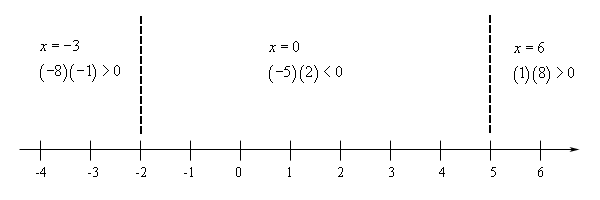Now, let’s talk about this a little. When we pick test points make sure that you pick easy numbers to work with. So, don’t choose large numbers or fractions unless you are forced to by the problem.

Also, note that we plugged the test points into the factored form of the polynomial and all we’re really after here is whether or not the polynomial is positive or negative. Therefore, we didn’t actually bother with values of the polynomial just the sign and we can get that from the product shown. The product of two negatives is a positive, etc.

We are now ready for the final step in the process.

Step 5 : Write down the answer. Recall that we discussed earlier that if any point from a region satisfied the inequality then ALL points in that region satisfied the inequality and likewise if any point from a region did not satisfy the inequality then NONE of the points in that region would satisfy the inequality.

This means that all we need to do is look up at the number line above. If the test point from a region satisfies the inequality then that region is part of the solution. If the test point doesn’t satisfy the inequality then that region isn’t part of the solution.

Now, also notice that any value of $$x$$ that will satisfy the original inequality will also satisfy the inequality from Step 2 and likewise, if an $$x$$ satisfies the inequality from Step 2 then it will satisfy the original inequality.

So, that means that all we need to do is determine the regions in which the polynomial from Step 2 is negative. For this problem that is only the middle region. The inequality and interval notation for the solution to this inequality are,

$- 2 < x < 5\hspace{0.5in}\left( { - 2,5} \right)$

Notice that we do need to exclude the endpoints since we have a strict inequality (< in this case) in the inequality.

Okay, that seems like a long process, however, it really isn’t. There was lots of explanation in the previous example. The remaining examples won’t be as long because we won’t need quite as much explanation in them.

Example 2 Solve $${x^2} - 5x \ge - 6$$.
Show Solution

Okay, this time we’ll just go through the process without all the explanations and steps. The first thing to do is get a zero on one side and factor the polynomial if possible.

$\begin{array}{c}{x^2} - 5x + 6 \ge 0\\ \left( {x - 3} \right)\left( {x - 2} \right) \ge 0\end{array}$

So, the polynomial will be zero at$$x = 2$$ and $$x = 3$$. Notice as well that unlike the previous example, these will be solutions to the inequality since we’ve got a “greater than or equal to” in the inequality.

Here is the number line for this example.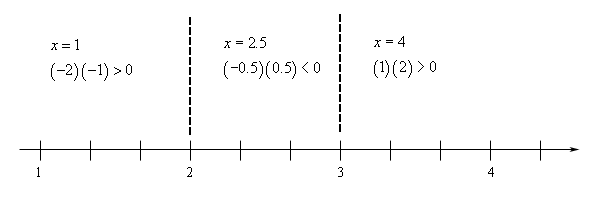Notice that in this case we were forced to choose a decimal for one of the test points.

Now, we want regions where the polynomial will be positive. So, the first and last regions will be part of the solution. Also, in this case, we’ve got an “or equal to” in the inequality and so we’ll need to include the endpoints in our solution since at this points we get zero for the inequality and $$0 \ge 0$$ is a true inequality.

Here is the solution in both inequality and interval notation form.

$\begin{array}{c} - \infty < x \le 2\hspace{0.25in}{\mbox{and}}\hspace{0.25in}3 \le x < \infty \\ \left( { - \infty ,2} \right]\hspace{0.25in}{\mbox{and}}\hspace{0.25in}\left[ {3,\infty } \right)\end{array}$
Example 3 Solve $${x^4} + 4{x^3} - 12{x^2} \le 0$$.
Show Solution

Again, we’ll just jump right into the problem. We’ve already got zero on one side so we can go straight to factoring.

$\begin{array}{c}{x^4} + 4{x^3} - 12{x^2} \le 0\\ {x^2}\left( {{x^2} + 4x - 12} \right) \le 0\\ {x^2}\left( {x + 6} \right)\left( {x - 2} \right) \le 0\end{array}$

So, this polynomial is zero at $$x = - 6$$, $$x = 0$$ and $$x = 2$$. Here is the number line for this problem.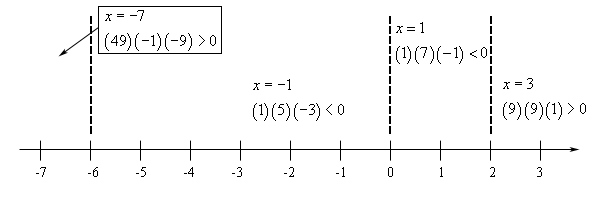First, notice that unlike the first two examples these regions do NOT alternate between positive and negative. This is a common mistake that students make. You really do need to plug in test points from each region. Don’t ever just plug in for the first region and then assume that the other regions will alternate from that point.

Now, for our solution we want regions where the polynomial will be negative (that’s the middle two here) or zero (that’s all three points that divide the regions). So, we can combine up the middle two regions and the three points into a single inequality in this case. The solution, in both inequality and interval notation form, is.

$- 6 \le x \le 2\hspace{0.25in}\hspace{0.25in}\left[ { - 6,2} \right]$
Example 4 Solve $$\left( {x + 1} \right){\left( {x - 3} \right)^2} > 0$$.
Show Solution

The first couple of steps have already been done for us here. So, we can just straight into the work. This polynomial will be zero at $$x = - 1$$ and $$x = 3$$. Here is the number line for this problem.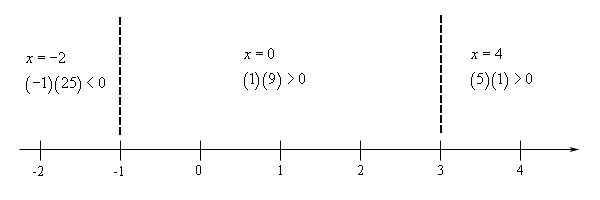Again, note that the regions don’t alternate in sign!

For our solution to this inequality we are looking for regions where the polynomial is positive (that’s the last two in this case), however we don’t want values where the polynomial is zero this time since we’ve got a strict inequality (>) in this problem. This means that we want the last two regions, but not $$x = 3$$.

So, unlike the previous example we can’t just combine up the two regions into a single inequality since that would include a point that isn’t part of the solution. Here is the solution for this problem.

$\begin{array}{c} - 1 < x < 3\hspace{0.25in}{\mbox{and}}\hspace{0.25in}3 < x < \infty \\ \left( { - 1,3} \right)\hspace{0.25in}{\mbox{and}}\hspace{0.25in}\left( {3,\infty } \right)\end{array}$

Now, all of the examples that we’ve worked to this point involved factorable polynomials. However, that doesn’t have to be the case. We can work these inequalities even if the polynomial doesn’t factor. We should work one of these just to show you how they work.

Example 5 Solve $$3{x^2} - 2x - 11 > 0$$.
Show Solution

In this case the polynomial doesn’t factor so we can’t do that step. However, we do still need to know where the polynomial is zero. We will have to use the quadratic formula for that. Here is what the quadratic formula gives us.

$x = \frac{{1 \pm \sqrt {34} }}{3}$

In order to work the problem we’ll need to reduce this to decimals.

$x = \frac{{1 + \sqrt {34} }}{3} = 2.27698\hspace{0.5in}x = \frac{{1 - \sqrt {34} }}{3} = - 1.61032$

From this point on the process is identical to the previous examples. In the number line below the dashed lines are at the approximate values of the two decimals above and the inequalities show the value of the quadratic evaluated at the test points shown.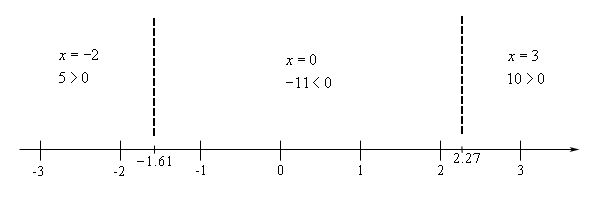So, it looks like we need the two outer regions for the solution. Here is the inequality and interval notation for the solution.

$\begin{array}{c} \displaystyle - \infty < x < \frac{{1 - \sqrt {34} }}{3}\hspace{0.25in}{\mbox{and}}\hspace{0.25in}\frac{{1 + \sqrt {34} }}{3} < x < \infty \\ \displaystyle \left( { - \infty ,\frac{{1 - \sqrt {34} }}{3}} \right)\hspace{0.25in}{\mbox{and}}\hspace{0.25in}\left( {\frac{{1 + \sqrt {34} }}{3},\infty } \right)\end{array}$